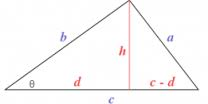Search IntMath
Close

# Graphical question [Solved!]

### My questionIn the above given triangle when theta = 0 then b=c
But when theta = 90 then d=0
Since d is the projection of b
How we can derive a generalised formula for d or c-d with respect to theta
Plese may kindly be elaborated.

### Relevant page

1. Distance Formula

### What I've done so far

X

<img src="/forum/uploads/imf-3408-triangle-question-image2.png" width="208" height="104" alt="trigonometric-graphs" />

In the above given triangle when theta = 0 then b=c
But when theta  = 90 then d=0
Since d is the projection of b
How we can derive a generalised formula for d or c-d with respect to theta
Plese may kindly be elaborated.
Relevant page

<a href="/plane-analytic-geometry/1-distance-formula.php">1. Distance Formula</a>

What I've done so far



## Re: Graphical question

Hello mfaisal

I can't see any working, so I'm not sure where you are getting stuck. Always show some steps so we can better help you.

The question seems a bit strange to me. Surely if b=c. then it won't matter what the angle theta is?

X

Hello mfaisal

I can't see any working, so I'm not sure where you are getting stuck. Always show some steps so we can better help you.

The question seems a bit strange to me. Surely if b=c. then it won't matter what the angle theta is?

## Re: Graphical question

A generalised formula would need to use trigonometry, I believe, something like

tan theta = h/d

I'm not sure there is any more "generalised" way to answer it.

X

mfaisal did not reply.

A generalised formula would need to use trigonometry, I believe, something like

tan theta = h/d

I'm not sure there is any more "generalised" way to answer it.